## In answering a question on a multiple choice test for class XII, a student either knows the answer or guesses. Let 3/5 be the probability that he knows the answer and 2/5 be the probability that he guesses. Assume that a student who guesses at the answer will be correct with probability 1/5. Let E 1 , E 2 , E be the events that the student knows the answer, guesses the answer and answers correctly respectively.(adsbygoogle = window.adsbygoogle || []).push({}); Based on the above information, answer the following: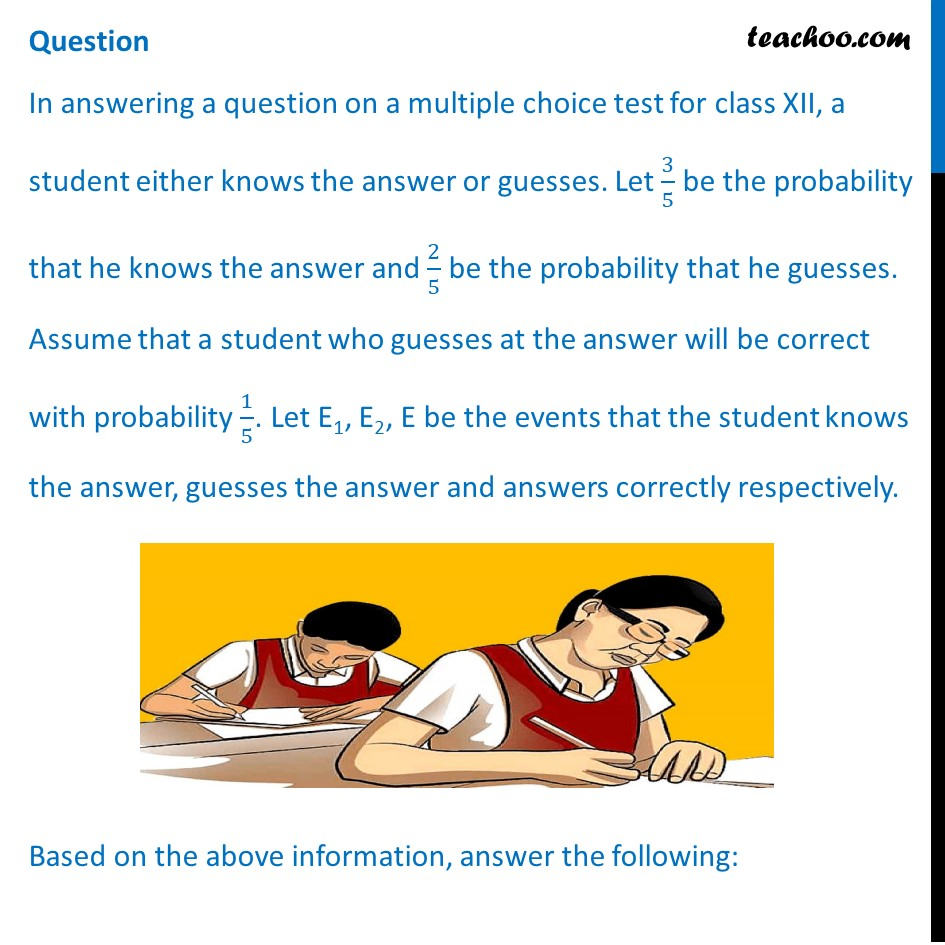## (d) 3/5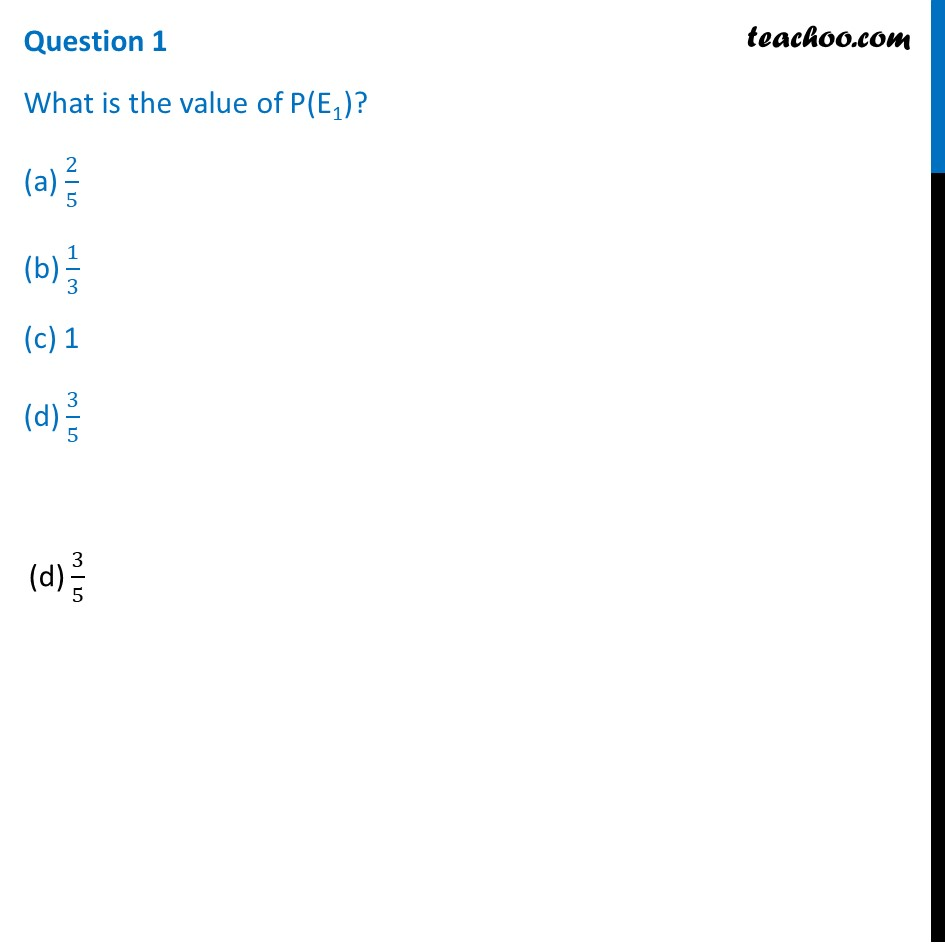## (d) 415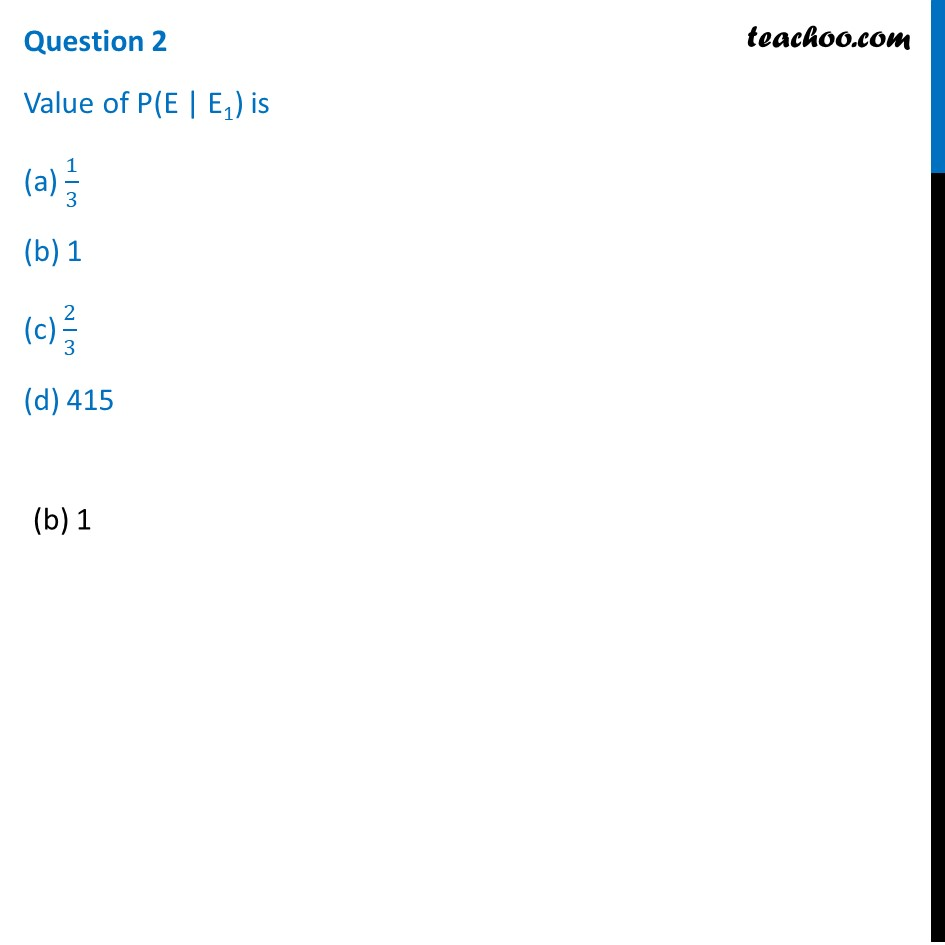## (d) 1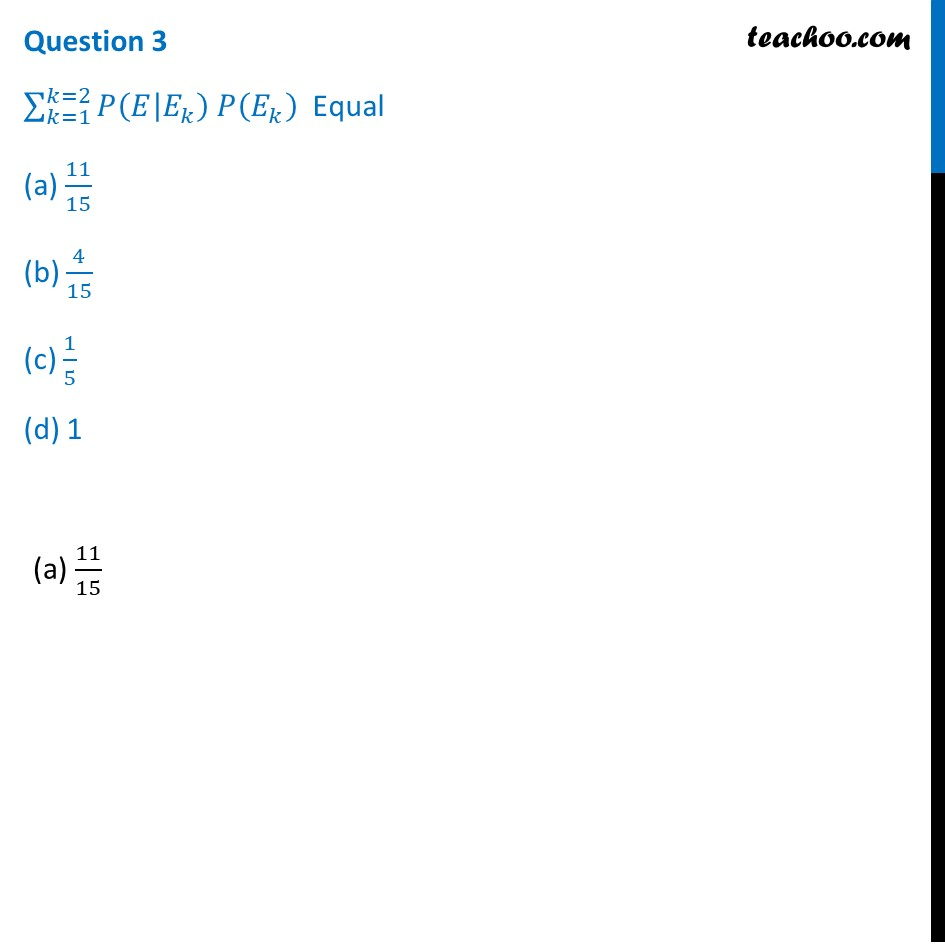## (d) 3/5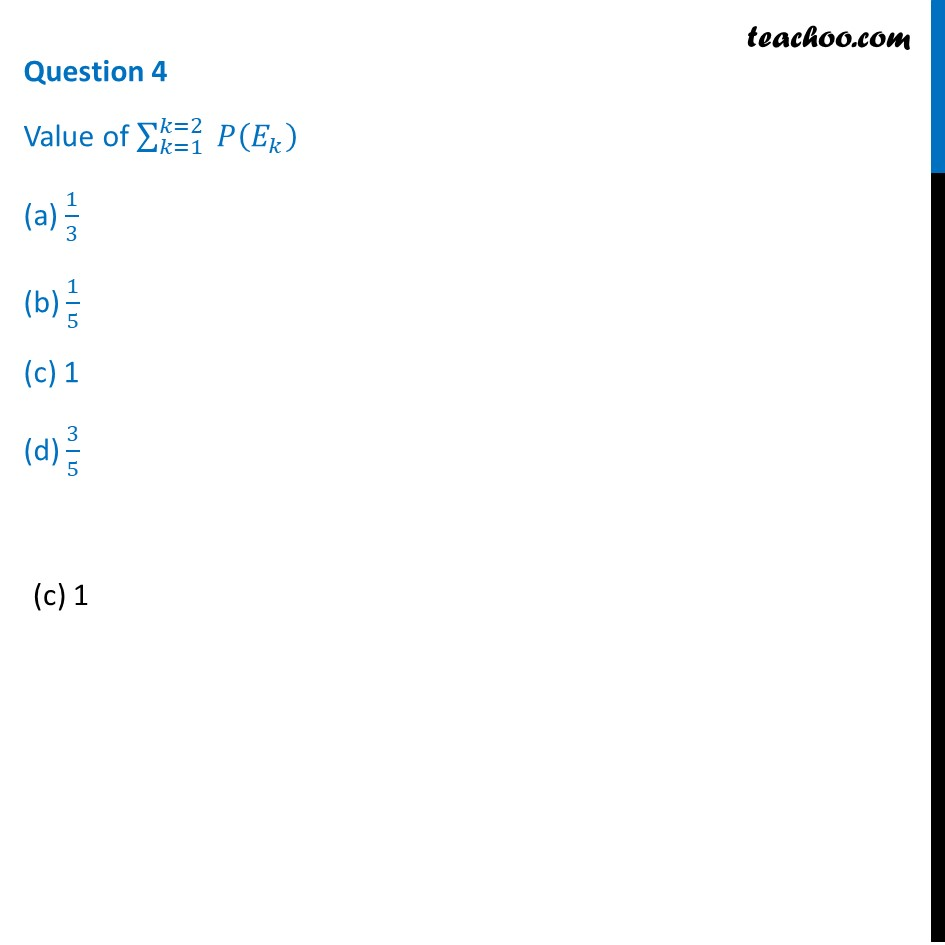## (d) 13/3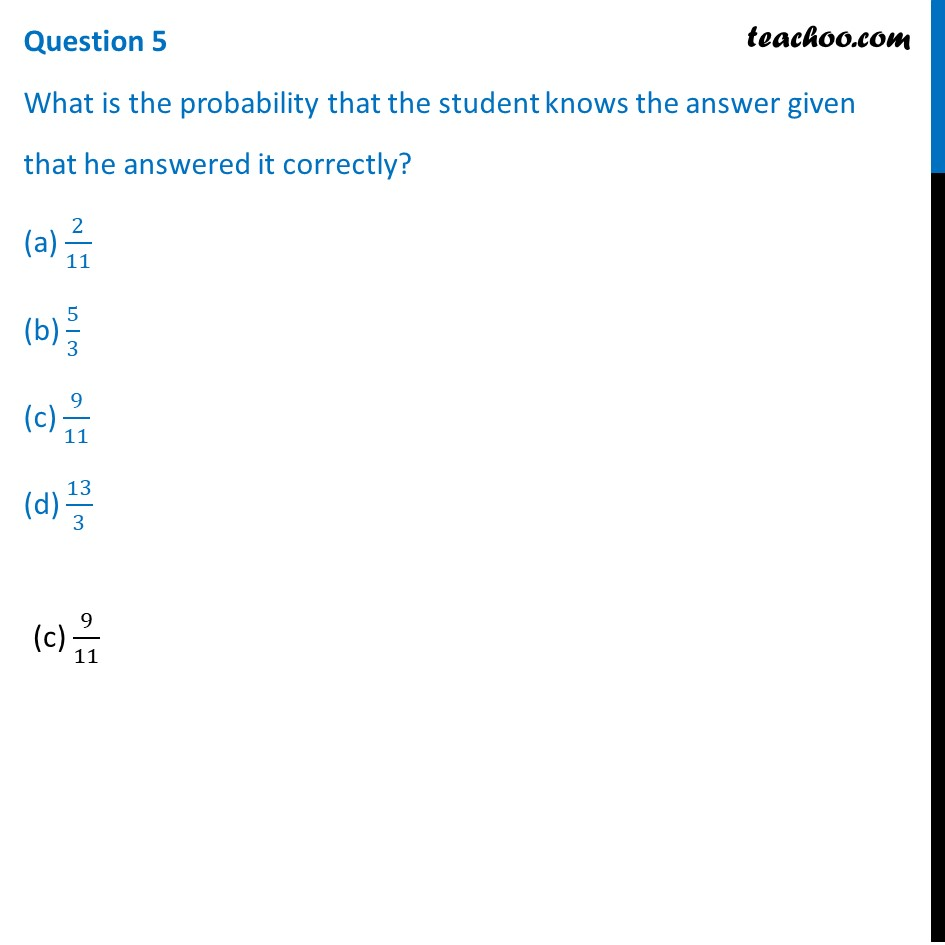1. Chapter 13 Class 12 Probability (Term 2)
2. Serial order wise
3. Case Based Questions (MCQ)

Transcript

In answering a question on a multiple choice test for class XII, a student either knows the answer or guesses. Let 3/5 be the probability that he knows the answer and 2/5 be the probability that he guesses. Assume that a student who guesses at the answer will be correct with probability 1/5. Let E1, E2, E be the events that the student knows the answer, guesses the answer and answers correctly respectively. Based on the above information, answer the following: Question 1 What is the value of P(E1)? (a) 2/5 (b) 1/3 (c) 1 (d) 3/5(d) 3/5 Question 2 Value of P(E | E1) is (a) 1/3 (b) 1 (c) 2/3 (d) 415 (b) 1 Question 3 ∑_(𝑘=1)^(𝑘=2)▒〖𝑃(𝐸│𝐸_𝑘 ) 𝑃(𝐸_𝑘 ) 〗 Equal (a) 11/15 (b) 4/15 (c) 1/5 (d) 1 (a) 11/15 Question 4 Value of ∑_(𝑘=1)^(𝑘=2)▒〖 𝑃(𝐸_𝑘 ) 〗 (a) 1/3 (b) 1/5 (c) 1 (d) 3/5(c) 1 Question 5 What is the probability that the student knows the answer given that he answered it correctly? (a) 2/11 (b) 5/3 (c) 9/11 (d) 13/3(c) 9/11

Case Based Questions (MCQ)